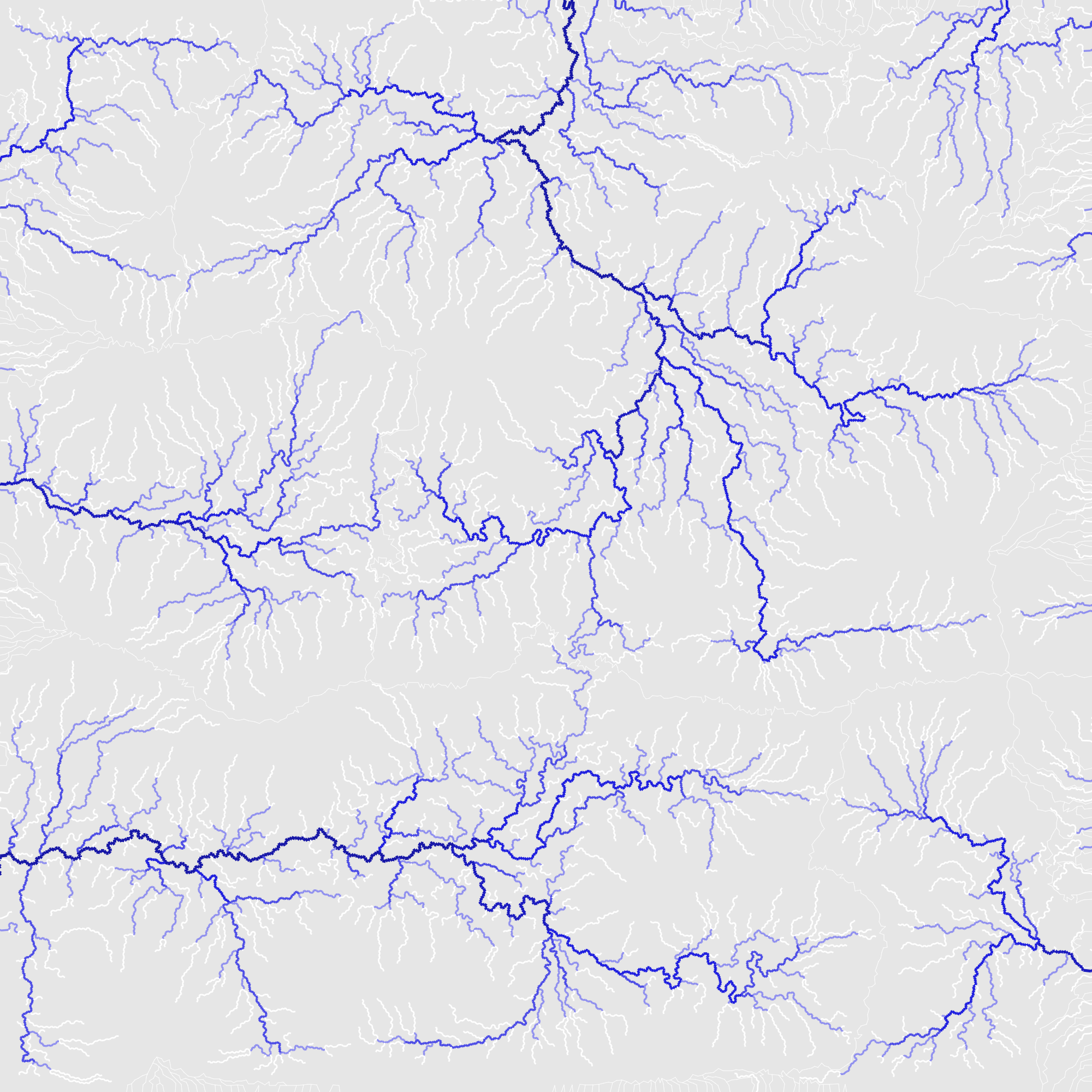# Distance to Selected Points

from Red Blob Games
Apr 2014, updated Feb 2015, May 2020

Running Breadth First Search with multiple start points can do some cool things.

## Distance to nearest obstacle#

Nairou on StackExchange asked how to calculate the distance to the nearest obstacle in a single pass. One answer is Breadth First Search. Usually in pathfinding we initialize the open set with a single start point, but when calculating the distance to the nearest obstacle, we consider each obstacle a start point. Here’s a quick & dirty demo. Click to cycle among: open space (blue), start point (purple), and wall (gray)

Here’s some Python code:

```frontier = Queue()
cost_so_far = {}

# Set the distance to 0 at all start points
for s in start:
frontier.put(s)
cost_so_far[s] = 0

# Expand outwards from existing points
while not frontier.empty():
current = frontier.get()
for next in current.neighbors():
if next not in cost_so_far:
cost_so_far[next] = cost_so_far[current] + 1
frontier.put(next)```

## Distance to nearest intersection#

Here’s another demo, showing how it works in mazes (based on this). I marked intersections as start points (purple) and then marked entrances to the big room on the left, but you can mark anything you want:

## Distance to nearest wall#

Here’s the same algorithm with the walls as the start points. It tells you not only the size of the room but also how to move towards or away from the center. Click to toggle walls:

Walls, intersections, enemies, allies, resources, bosses, treasures — there are so many types of map analysis you might do with this. For example, corridors are 1, but doorways and other tiles have 1 also. If you mark all the tiles that are 1 and are surrounded by 0 or 1, those will be the corridors.

## Region growth#

If in addition to the distance, we keep track of which  start point (seed) that distance is based on, we end up with a map that tells us which start point is closest to each other point based on travel distance.

```frontier = Queue()
cost_so_far = {}
seed = {}

# Set the distance to 0 at all start points,
# and each start point is its own seed
for s in start:
frontier.put(s)
cost_so_far[s] = 0
seed[s] = s

# Expand outwards from existing points
while not frontier.empty():
current = frontier.get()
for next in current.neighbors():
if next not in cost_so_far:
cost_so_far[next] = cost_so_far[current] + 1
seed[next] = seed[current]
frontier.put(next)```

Run it again a second time, this time with the country borders as the start points, and you can find paths towards / away from the borders.

## More ideas#

The same algorithm works on a polygon mesh. Switch to Dijkstra’s Algorithm to extend this to work with variable movement costs. Take a look at this article for more ideas.

There are other algorithms, some of them more easily parallelizable or GPU-friendly than Breadth First Search. See Distance Transform on Wikipedia. For Euclidean distance instead of Manhattan Distance, see 2D Euclidean Distance Transforms: A Comparative Study (PDF).

1. In a map generation experiment I started at the coastlines and used Breadth First Search with some randomness to generate rivers.Breadth First Search, growing rivers from the coast